SSC  >  SSC CPO Mock Test (Paper 1) - 5

# SSC CPO Mock Test (Paper 1) - 5

Test Description

## 200 Questions MCQ Test SSC CPO & Constable - Mock Tests & Previous Year Papers | SSC CPO Mock Test (Paper 1) - 5

SSC CPO Mock Test (Paper 1) - 5 for SSC 2023 is part of SSC CPO & Constable - Mock Tests & Previous Year Papers preparation. The SSC CPO Mock Test (Paper 1) - 5 questions and answers have been prepared according to the SSC exam syllabus.The SSC CPO Mock Test (Paper 1) - 5 MCQs are made for SSC 2023 Exam. Find important definitions, questions, notes, meanings, examples, exercises, MCQs and online tests for SSC CPO Mock Test (Paper 1) - 5 below.
Solutions of SSC CPO Mock Test (Paper 1) - 5 questions in English are available as part of our SSC CPO & Constable - Mock Tests & Previous Year Papers for SSC & SSC CPO Mock Test (Paper 1) - 5 solutions in Hindi for SSC CPO & Constable - Mock Tests & Previous Year Papers course. Download more important topics, notes, lectures and mock test series for SSC Exam by signing up for free. Attempt SSC CPO Mock Test (Paper 1) - 5 | 200 questions in 120 minutes | Mock test for SSC preparation | Free important questions MCQ to study SSC CPO & Constable - Mock Tests & Previous Year Papers for SSC Exam | Download free PDF with solutions
 1 Crore+ students have signed up on EduRev. Have you?
SSC CPO Mock Test (Paper 1) - 5 - Question 1

### Complete the analogous pair.CDBE : EFDG :: NOMP : ?

SSC CPO Mock Test (Paper 1) - 5 - Question 2

### Re-arrange the given words in alphabetical order and tick the one that comes in the middle.

SSC CPO Mock Test (Paper 1) - 5 - Question 3

### Find out which word or name will be in the middle after the words or names are arranged in the alphabetical order.

SSC CPO Mock Test (Paper 1) - 5 - Question 4
Choose the group of letters which is different from others.
SSC CPO Mock Test (Paper 1) - 5 - Question 5
Choose the word which is least like the other words in the group .
SSC CPO Mock Test (Paper 1) - 5 - Question 6
Choose the word which is least like the other words in the group .
SSC CPO Mock Test (Paper 1) - 5 - Question 7
Complete the analogous pair.
Cricket : Bat : : Hockey : ?
SSC CPO Mock Test (Paper 1) - 5 - Question 8
Complete the analogous pair.
Cenotaph : Cemetary :: Corpse : ?
SSC CPO Mock Test (Paper 1) - 5 - Question 9
Complete the analogous pair.
Car : Petrol : : Television : ?
SSC CPO Mock Test (Paper 1) - 5 - Question 10
Complete the analogous pair.
Ship : Sea : : Camel :?
SSC CPO Mock Test (Paper 1) - 5 - Question 11
A child crawls 20 feet towards North, turns right and crawls 30 feet, turns right again and crawls 35 feet. He turns left now and crawls 15 feet. He turns left again and crawls 15 feet. Finally he turns to his left to crawl another 15 feet. How far is he from his starting point and in which direction?
SSC CPO Mock Test (Paper 1) - 5 - Question 12
How many squares are there in the following figure?
Detailed Solution for SSC CPO Mock Test (Paper 1) - 5 - Question 12 There are a total of nineteen squares in the figure,
which have been marked as shown in the following figure

There are three squares in the upper row EFIH, FGJI and GBLJ
There are five squares in the middle row - TQRS, QHPR, HIOP, IJNO and JLMN
There are three squares in the lower row - SRUD, RPVU, POWV
There are a total of six squares three in each of the two rectangles - ABMS, TLCD, AEPS, EGNP, FBMO, THVD, QIWU, ILCW
There are two squares in the main rectangle ABCD - AFWD, EBCV
SSC CPO Mock Test (Paper 1) - 5 - Question 13
If South-east becomes North, North-east becomes West and so on, what will West become ?
Detailed Solution for SSC CPO Mock Test (Paper 1) - 5 - Question 13
From the diagrams that new name of West will become South-East
SSC CPO Mock Test (Paper 1) - 5 - Question 14
If first half of the following series is written in the reverse order, which will be the 5th letter to the left of 13th letter from your right?
ABCDEFGHIJKLMNOPQRSTUVWXYZ
SSC CPO Mock Test (Paper 1) - 5 - Question 15
Find the missing character.
Detailed Solution for SSC CPO Mock Test (Paper 1) - 5 - Question 15 The lower number is the difference of the squares of the upper two numbers. Thus,
In fig. (A), 122-82=80.
In fig. (B), 162-72=207.
$\therefore$In fig. (C), missing number = 252-212=625-441=184.
SSC CPO Mock Test (Paper 1) - 5 - Question 16
Group the following figures into three classes on the basis of identical properties.
Detailed Solution for SSC CPO Mock Test (Paper 1) - 5 - Question 16 Image 1, 3 and 5 are circle related
Image 2, 6, 8 are triangle
Image related 4, 7, 9 are quadrilateral
SSC CPO Mock Test (Paper 1) - 5 - Question 17
Find the missing character.
SSC CPO Mock Test (Paper 1) - 5 - Question 18
Find the missing character.
SSC CPO Mock Test (Paper 1) - 5 - Question 19

Choose the odd one out.

SSC CPO Mock Test (Paper 1) - 5 - Question 20
Choose the odd one out.
SSC CPO Mock Test (Paper 1) - 5 - Question 21

How many such pairs of letters are there in the word CONTROL each of which has as many letters between them in the word as in the English alphabet ?

Detailed Solution for SSC CPO Mock Test (Paper 1) - 5 - Question 21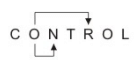SSC CPO Mock Test (Paper 1) - 5 - Question 22
In a coded language Q U A L I T A T I V E is written as T A U L A T I T E V. How is
L O G I C A L written in the same language:-
SSC CPO Mock Test (Paper 1) - 5 - Question 23

In a certain code 'DOUBT is written as 53146' and 'TEAM is written as 6972'. How is ABODE wirtten in that code ?

Detailed Solution for SSC CPO Mock Test (Paper 1) - 5 - Question 23

A → 7, B → 4,

O → 3,

D → 5, E → 9

Check from the codes given for other words in the question

SSC CPO Mock Test (Paper 1) - 5 - Question 24
Which of the following combination of figures best represents Liquid, Milk and River water?
SSC CPO Mock Test (Paper 1) - 5 - Question 25

Study the following diagram :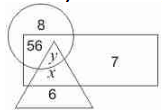The value of (x + y) is

Detailed Solution for SSC CPO Mock Test (Paper 1) - 5 - Question 25

56 = 8 x 7
x = 6 x 7 = 42
∴ y = 8 x 42 = 336
∴ x + y = 336 + 42 = 378

SSC CPO Mock Test (Paper 1) - 5 - Question 26

If A means 'x', B means '÷', C means '-' and D means '+' then -
4 D 16 A 5 B 8 C 5 = ?

Detailed Solution for SSC CPO Mock Test (Paper 1) - 5 - Question 26

4 D 16 A 5 B 8 C 5
= 4 + 16 x 5 x 8 - 5 (Use BODMAS Rule)
= 4 + 10 - 5
= 9

SSC CPO Mock Test (Paper 1) - 5 - Question 27
Complete the analogous pair.
211 : 333 :: 356 : ?
Detailed Solution for SSC CPO Mock Test (Paper 1) - 5 - Question 27 Addition of sum of digits of 2nd term (333) and 3rd term (356) = 9 + 14 = 23
Again addition of sum of digits of 1st term (211) and option (A)(358) = 4 + 16 ≠ 23
Again addition of sum of digits of 1st term (211) and option (B)(329) = 4 + 14 ≠ 23
Again addition of sum of digits of 1st term (211) and option (C)(423) = 4 + 9 ≠ 23
Again addition of sum of digits of 1st term (211) and option (D)(388) = 4 + 19 = 23
SSC CPO Mock Test (Paper 1) - 5 - Question 28
Choose the odd one out.
SSC CPO Mock Test (Paper 1) - 5 - Question 29
Choose the odd one out.
SSC CPO Mock Test (Paper 1) - 5 - Question 30
Choose the odd one out.
SSC CPO Mock Test (Paper 1) - 5 - Question 31
Find out from amongst the four alternatives as to how the pattern would appear when the transparent sheet is folded at the dotted line.
SSC CPO Mock Test (Paper 1) - 5 - Question 32
Find the wrong number in the series.
7, 9, 16, 25, 41, 68, 107, 173
Detailed Solution for SSC CPO Mock Test (Paper 1) - 5 - Question 32 The series is 7+9=16;
16+9=25
25+16=41;
41+25=66;
66+41=107,.........
SSC CPO Mock Test (Paper 1) - 5 - Question 33
Choose the correct alternative that will continue the same pattern and fill in the blank.
19, 2, 38, 3, 114, 4, (....)
SSC CPO Mock Test (Paper 1) - 5 - Question 34
Use the following series of elements (alpha number symbol) to answer these questions. Every two digit number (given in brackets) is to be treated as single number.
2 * 8 5 6 B 9 \$ Q 3 E 1 7 R D 4 (13) U∙ K (18) A (14) P
If each of the letters in the above series of elements is given a value equivalent to its serial number in the English alphabet, what will the difference between the sum of the consonants and the sum of the vowels used in the series?
SSC CPO Mock Test (Paper 1) - 5 - Question 35
In this letter series, some of the letters are missing. Choose the correct letter given below -
_ _ babbba _ a _ _
SSC CPO Mock Test (Paper 1) - 5 - Question 36
Find out the term in the number series which is wrong.
196, 169, 144, 121, 80
SSC CPO Mock Test (Paper 1) - 5 - Question 37

Find the wrong number in the series.
27 -> 12, 12 -> 8, 33-> 14,

Detailed Solution for SSC CPO Mock Test (Paper 1) - 5 - Question 37

12x2+3 ->12
4x2+4 ->4
14x2+5 ->14

SSC CPO Mock Test (Paper 1) - 5 - Question 38
In each question a statement followed by two assumptions numbered I and II is given. Consider the statement and decide which of the given assumption is implicit.
Statement : Sachin wrote to his brother at Bangalore to collect personally the application form from the University for the post-graduation course in Mathematics.
Assumptions : I. The University may issue application forms to a person other than the prospective student.
II. Sachin's brother may receive the letter well before the last date of collecting application forms.
SSC CPO Mock Test (Paper 1) - 5 - Question 39
Below is given statement followed by two conclusions numbered I and II. You have to consider the statement and the following conclusions and decide which of the conclusions is follows in the statement :
Statements :a. All books are pens.
b. Some pens are desks.
c. Some desks are chairs.
d. Some chairs are tables.
conclusions:I. Some tables are desks.
II. Some chairs are pens.
SSC CPO Mock Test (Paper 1) - 5 - Question 40
If Rajdoot is coded as Car, Car as Aeroplane, Aeroplane as Train, Train as Bus, then by which vehicle can you reach your destination in least time? .
SSC CPO Mock Test (Paper 1) - 5 - Question 41
In each question, five words are given. Four of them are alike in some way. One is different from them.Which is the one that does not belong to that group?
SSC CPO Mock Test (Paper 1) - 5 - Question 42
Bus for Chikodi village is after every hour from 7.30 am to 12.30 pm but after that it is after every one and half hours gap. One bus has gone half an hour ago, Vilas was told by a depot clerk at 5.30 pm. When was the next bus scheduled ?
SSC CPO Mock Test (Paper 1) - 5 - Question 43
Choose one word which cannot be made from the letters of the given word
ENDEAVOUR
SSC CPO Mock Test (Paper 1) - 5 - Question 44

In the following questions, select a figure from amongst the four alternatives, which when placed in the blank space of fig. (X) would complete the pattern.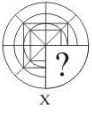SSC CPO Mock Test (Paper 1) - 5 - Question 45

In each question below is given a group of letters followed by four combinations of digits numbered (A), (B), (C) and (D). You have to find put which of the combinations correctly represents the group of letters based on the following coding system and mark the number of that combination as the answer. If none of the four combinations correctly represents the group of letters, mark (E) i.e. 'None of these' as the answer.
Letter : B L C X P D S K M E
Digits :  7  1  0  8 9  3 6  2  5  4

KCDEBPS

SSC CPO Mock Test (Paper 1) - 5 - Question 46

In each of the following questions, which one of the for interchanges in signs and numbers would make the given equation correct?

29. 6 × 4 + 2 = 16

Detailed Solution for SSC CPO Mock Test (Paper 1) - 5 - Question 46

On interchanging + and × and 4 and 6, we get the equation as 4 + 6 × 2 = 16 or 4 + 12 = 16 or 16 = 16, which is true

SSC CPO Mock Test (Paper 1) - 5 - Question 47

In each of the following questions, which one of the for interchanges in signs and numbers would make the given equation correct?

30. (3 ÷ 4) + 2 = 2

Detailed Solution for SSC CPO Mock Test (Paper 1) - 5 - Question 47

On interchanging + and ÷ and 2 and 3, we get the equation as (2 + 4) ÷ 3 = 2 or 6 ÷ 3 = 2 or 2 = 2, which is true

SSC CPO Mock Test (Paper 1) - 5 - Question 48

Select the correct mirror image of the figure (X) from amongst the given alternatives.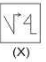SSC CPO Mock Test (Paper 1) - 5 - Question 49

Each of the following question consist of five problem figure marked 1, 2, 3, 4, 5 followed by Answer figure A, B, C, D and E. Select of figure from the answer figure which will continue the same series as given in the problem figure.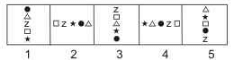SSC CPO Mock Test (Paper 1) - 5 - Question 50

In the questions, figure (X) is embedded in one of following figures. Find out the correct alternative.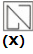SSC CPO Mock Test (Paper 1) - 5 - Question 51

The test of 'reasonableness' of a law depriving a person of the freedoms of Art.19 was also made applicable to Art.21 in which case?

SSC CPO Mock Test (Paper 1) - 5 - Question 52

Which state has become the most densely populated state with 1102 persons per square kilometer?

SSC CPO Mock Test (Paper 1) - 5 - Question 53

The basic principle of Parliamentary Government is-

SSC CPO Mock Test (Paper 1) - 5 - Question 54

Which article incorporates the concept of judicial review?

SSC CPO Mock Test (Paper 1) - 5 - Question 55

Who started the first newspaper in India?

SSC CPO Mock Test (Paper 1) - 5 - Question 56

Which of the following floppy sizes exists?

SSC CPO Mock Test (Paper 1) - 5 - Question 57

Which goods is not imported in India ?

SSC CPO Mock Test (Paper 1) - 5 - Question 58

The main difference between application and system software is that:

SSC CPO Mock Test (Paper 1) - 5 - Question 59

Which of the folowing Industries employs the largest number of workers in India?

SSC CPO Mock Test (Paper 1) - 5 - Question 60

"Open market operation" is a part of-

SSC CPO Mock Test (Paper 1) - 5 - Question 61

Which plan gave emphasis on removal of poverty for the first time?

SSC CPO Mock Test (Paper 1) - 5 - Question 62

Limited liability means :

SSC CPO Mock Test (Paper 1) - 5 - Question 63

Which is first public sector unit in India to be disinvested?

SSC CPO Mock Test (Paper 1) - 5 - Question 64

In India those farmers are called ‘Marginal Farmers’ who hold the land upto—

SSC CPO Mock Test (Paper 1) - 5 - Question 65

The concept of supply curve as it is used in economic theory is relevant only for the case of-

SSC CPO Mock Test (Paper 1) - 5 - Question 66

Where is the Sahara Desert?

SSC CPO Mock Test (Paper 1) - 5 - Question 67

The largest natural satellite, Titan, is around which planet?

SSC CPO Mock Test (Paper 1) - 5 - Question 68

The Netherlands is the new name of

SSC CPO Mock Test (Paper 1) - 5 - Question 69

Which one of the following pairs is correctly matched ?

SSC CPO Mock Test (Paper 1) - 5 - Question 70

Who among the following is credited with the authorship of the Periplus of the Erythraean Sea ?

SSC CPO Mock Test (Paper 1) - 5 - Question 71

The strait of malacca lies between—

SSC CPO Mock Test (Paper 1) - 5 - Question 72

What lead Nawab Sirajuddaulah to attack Fort William in Calcutta in 1756

SSC CPO Mock Test (Paper 1) - 5 - Question 73

The ultimate source of law according to Vedlic philosophy was

SSC CPO Mock Test (Paper 1) - 5 - Question 74

What does Bhagwat Gita primarily deal with

SSC CPO Mock Test (Paper 1) - 5 - Question 75

The Swaraj Party was established in -

SSC CPO Mock Test (Paper 1) - 5 - Question 76

In which among the following molecules is the distance between two adjacent carbon atoms largest?

SSC CPO Mock Test (Paper 1) - 5 - Question 77

Who is considered the hero of Mahabharata ?

SSC CPO Mock Test (Paper 1) - 5 - Question 78

A tree species in Mauritius failed to reproduce because of the extinction of a fruit-eating bird. Which one of the following was that bird?

SSC CPO Mock Test (Paper 1) - 5 - Question 79

According to the World Health Organisation the disease which causes the death of the largest number of people today is:

SSC CPO Mock Test (Paper 1) - 5 - Question 80

People drinking water from a shallow handpump are likely to suffer from all of the following diseases except

SSC CPO Mock Test (Paper 1) - 5 - Question 81

Assertion [A] : Artificial satellites are always launched from the earth in the eastward direction.
Reason [R] : The earth rotates from west to east and so the satellite attains the escape velocity.

SSC CPO Mock Test (Paper 1) - 5 - Question 82

Which one of the following pairs of materials serves as electrodes in chargeable batteries commonly used in devices such as torch lights, electric shavers etc.?

SSC CPO Mock Test (Paper 1) - 5 - Question 83

Assertion [A] : In a motion picture, usually 24 frames are projected every second over the whole length of the film.
Reason [R] : An image formed on the retina of eye persists for about 0.1 s after the removal of stimulus.

SSC CPO Mock Test (Paper 1) - 5 - Question 84

In the case of a 'Test-tube bady'

SSC CPO Mock Test (Paper 1) - 5 - Question 85

In a bisexual flower, if androceium and gynaecium mature at different times, the phenomenon is known as

SSC CPO Mock Test (Paper 1) - 5 - Question 86

Who has been sworn in as the Deputy National Security Adviser (NSA) by the Union Government?

SSC CPO Mock Test (Paper 1) - 5 - Question 87

Polio is a disease caused due to :

SSC CPO Mock Test (Paper 1) - 5 - Question 88

Air-pockets that cause aircrafts to lose altitude suddenly are caused by

SSC CPO Mock Test (Paper 1) - 5 - Question 89

By opening the door of a refrigerator which is inside the room

SSC CPO Mock Test (Paper 1) - 5 - Question 90

Which animal fights snakes ?

SSC CPO Mock Test (Paper 1) - 5 - Question 91

Which of the following features distinguish musical sounds from noise ? (1) Regularity in sound (2) A musical sound has a single frequency (3) Absence of sudden changes of amplitude in respect of musical sounds

SSC CPO Mock Test (Paper 1) - 5 - Question 92

How do the centripetal forces pull a body ?

SSC CPO Mock Test (Paper 1) - 5 - Question 93

Greenhouse is

SSC CPO Mock Test (Paper 1) - 5 - Question 94

Who among the following has recently been appointed as the Director-General of Sports Authority of India (SAI)?

SSC CPO Mock Test (Paper 1) - 5 - Question 95

In which of the following way the age of a tree can be determined ?

SSC CPO Mock Test (Paper 1) - 5 - Question 96

Optical fibre works on the principle of

SSC CPO Mock Test (Paper 1) - 5 - Question 97

The Commonwealth completed its 50 yrs in

SSC CPO Mock Test (Paper 1) - 5 - Question 98

Which of the following is a language ?

SSC CPO Mock Test (Paper 1) - 5 - Question 99

What is the number of non-permanent members of the Security Council?

SSC CPO Mock Test (Paper 1) - 5 - Question 100

Which among the following is not a Asian country selected to the UN Committee on Human Rights for three year term beginning January, 1995?

SSC CPO Mock Test (Paper 1) - 5 - Question 101

Ravi gave Rs 1200 on loan. Some amount he gave at 4% per annum simple interest and remaining at 5% per annum simple interest. After two yrs, he got Rs 110 as interest. Then the amounts given at 4% and 5% per annum simple interest are,respectively,

SSC CPO Mock Test (Paper 1) - 5 - Question 102

If x + y = 6 and 3x - y = 4, then x - y is equal to

Detailed Solution for SSC CPO Mock Test (Paper 1) - 5 - Question 102

x + y = 6
⇒ y = 6 - x
Now, 3x - y = 4
or 3x - (6 - x) = 4
or 3x - 6 + x = 4
or 4x = 10
or x = 5 2
∴ y = 6 - 5/ 2 = 7 2
∴ x - y = 5/ 2 - 7 2 = -1

SSC CPO Mock Test (Paper 1) - 5 - Question 103

If 2 tables and 3 chairs cost Rs 2,315 and 2 chairs and 3 tables cost Rs 2,910,what is the cost of 4 chairs?

Detailed Solution for SSC CPO Mock Test (Paper 1) - 5 - Question 103

Let the cost of one table and chair be Rs x and Rs y
2x + 3y = 2315 .....(i)
3x + 2y = 2910 .....(ii)
Solving (i) and (ii) we get
y = 225, x = 820
∴ The cost of 4 chairs is Rs (4 x 225)
= Rs 900.00

SSC CPO Mock Test (Paper 1) - 5 - Question 104

Which one of the following is the solution of the system of linear equations (5/x)-(4/y)=3 and (9/x)-(8/y)=7?

SSC CPO Mock Test (Paper 1) - 5 - Question 105

The ratio of the age of a man and his wife is 4:3. After 4 yrs, this ratio will be 9:7. If at the time of the marriage, The ratio was 5:3, then how many yrs ago they were married?

Detailed Solution for SSC CPO Mock Test (Paper 1) - 5 - Question 105

4x + 4 3x + 4

= 9 7
or 28x + 28 = 27x + 36
or x = 8
Men's present age = 32yrs, Woman's age = 24 yrs
Let the required time be y years
32 - y 24 - y = 5 3 , y = 12 year

SSC CPO Mock Test (Paper 1) - 5 - Question 106

What is the ratio of the areas of two circles whose radii are in the ratio 1:2 ?

Detailed Solution for SSC CPO Mock Test (Paper 1) - 5 - Question 106

Let radius of one circle is x
So radius of other is 2x
Ratio of area = πx 2 π(2x) 2
= πx 2 /π4x 2 = 1 4 = 1 : 4

SSC CPO Mock Test (Paper 1) - 5 - Question 107

The ratio of two unequal sides of a rectangle is 1:2. If its perimeter is 24cm, then length of diagonal is

Detailed Solution for SSC CPO Mock Test (Paper 1) - 5 - Question 107

Let the sides of rectangle are x and 2x respectively. Then
2(x + 2x) = 24
⇒ x = 4
∴ Sides are 4 and 8 respectively.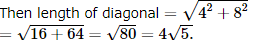SSC CPO Mock Test (Paper 1) - 5 - Question 108

A boy rides his bicycle 10 km at an average speed of 12 km/hr and again travel 12 km at an average speed of 10 km/hr. His average speed for the entire trip is approximately

SSC CPO Mock Test (Paper 1) - 5 - Question 109

The average of six numbers is x and the average of three of these is y. If the average of the remaining three is z, then:

Detailed Solution for SSC CPO Mock Test (Paper 1) - 5 - Question 109

x = (3y + 3z)/6
2x = y+ z

SSC CPO Mock Test (Paper 1) - 5 - Question 110

A bicycle wheel makes 500 revolutions in moving 1.1 km. The diameter of the wheel is

Detailed Solution for SSC CPO Mock Test (Paper 1) - 5 - Question 110

2πr = Circumference of the wheel = 1100 500 = 11 5
⇒ 2r = 11 / 5 x 7 /22 = 0.7 m

SSC CPO Mock Test (Paper 1) - 5 - Question 111

A TV and a VCR togehter cost Rs 35,000. If TV is 1 1/2 times costlier than VCR,then the cost of VCR is

Detailed Solution for SSC CPO Mock Test (Paper 1) - 5 - Question 111

Let cost of TV and VCR are Rs. x and Rs. y
Then x + y = Rs. 35000
Now, x = 3 2 y = 1.5y
1.5y + y = 35000
or, 2.5y = 35000
y = Rs. 14,000

SSC CPO Mock Test (Paper 1) - 5 - Question 112

The sum of the first 20 terms of the series (1/5x6)+(1/6x7)+(1/7x 8)+... is :

SSC CPO Mock Test (Paper 1) - 5 - Question 113

A ship at sea goes 60 km due east and then some distance due north. If now it is 61 km form its starting point then the distance travelled due north is

Detailed Solution for SSC CPO Mock Test (Paper 1) - 5 - Question 113

Using pythagorous theorem
x2 +602 = 612
x = 11 km

SSC CPO Mock Test (Paper 1) - 5 - Question 114

The vertices of a diagonal of a parallelogram are (3,-4) and (-6,5) and third vertex is (-2,1), then fourth vertex is

SSC CPO Mock Test (Paper 1) - 5 - Question 115

A finance company declares that, with Compound Interest, a sum of money deposited by anyone will become 8 times in 3 yrs. If the same amount is deposited at the same compound rate of interest, then in how many yrs it will become 16 times?

Detailed Solution for SSC CPO Mock Test (Paper 1) - 5 - Question 115

23 (8 times) → 3 years
24 (16 times) → ?
Total Number of years = 3 x 4 3 = 4 years

SSC CPO Mock Test (Paper 1) - 5 - Question 116

The cost price of an article is 64% of the marked price. Calculate the gain percent after allowing a discount of 12%.

Detailed Solution for SSC CPO Mock Test (Paper 1) - 5 - Question 116

Let marked price = Rs 100
Then C.P. = Rs 64. S.P = Rs 88
∴ Gain % = [ 24 64 x 100]% = 37.5%

SSC CPO Mock Test (Paper 1) - 5 - Question 117

Which of the following numbers is the least?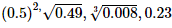SSC CPO Mock Test (Paper 1) - 5 - Question 118

Chords AB and CD of a circle when produced meet outside the circle at P. If AB = 4cm; BP = 3cm and CP = 14 cm. Then the length of CD is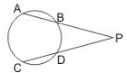Detailed Solution for SSC CPO Mock Test (Paper 1) - 5 - Question 118

Use PA x PB = PC x PD.

SSC CPO Mock Test (Paper 1) - 5 - Question 119

Locus of a point which is equidistant from two parallel line in a plane

SSC CPO Mock Test (Paper 1) - 5 - Question 120

The interior angles of a pentagon are in the ratio 1 : 2 : 3 : 5 : 9. The largest angle of the polygon is

Detailed Solution for SSC CPO Mock Test (Paper 1) - 5 - Question 120

Let angle be x
So, 1st angle is 1x
2nd angle is 2x
3rs angle is 3x
4th angle is 5x
& 5th angle is 9x
Sum of these angles is (1 + 2 + 3 + 5 + 9)x = 20x
Sum of the interior angle of a pentagon is = 540º
Hence x = 540 /20 = 27º
So, largest angle is 27 x 9 = 243º

SSC CPO Mock Test (Paper 1) - 5 - Question 121

The length of the shadow of a rod inclined at 10º to the vertical towards the sun is 2.05 metres when the elevation of the sun is 38º.The length of the rod is

SSC CPO Mock Test (Paper 1) - 5 - Question 122

In a division sum,a student took 42 as divisor instead of 24. His answer was 28.The correct answer must have been

SSC CPO Mock Test (Paper 1) - 5 - Question 123

The least perfect square number divisible by 2,3,4,6,8 is

SSC CPO Mock Test (Paper 1) - 5 - Question 124

Pipes A and B running together can fill a cistern in 6 minutes. If B takes 5 minutes more than A to fill the cistern, then the times in which A and B will fill the cistern separately will be respectively

SSC CPO Mock Test (Paper 1) - 5 - Question 125

The difference between a discount of 35% and two successive discounts of 20% on a certain bill was Rs.22. Find the amount of the bill.

SSC CPO Mock Test (Paper 1) - 5 - Question 126

65% of ? = 20% of 422.50

SSC CPO Mock Test (Paper 1) - 5 - Question 127

In a triangle ABC, A = 30º, b = 8, a = 6, then B = sin⁻1 x where x =

SSC CPO Mock Test (Paper 1) - 5 - Question 128

By selling 12 marbles for a rupee, a shopkeeper loses 20%. In order to gain 20% in the transaction, he should sell the marbles at the rate of how many marbles for a rupee?

Detailed Solution for SSC CPO Mock Test (Paper 1) - 5 - Question 128

Given : S.P. of 12 marbles / 0.8 = Rs. 1.25
Now S.P. of 12 marbles at a gain of 20%
= C.P. x 1.2 = 1.25 x 1.2 = Rs. 1.5
It means in order to gain 20% he should sell 12 marbles for Rs. 1.5. Or for Re . 1 he should sell 12 /1.5 = 8 marbles.

SSC CPO Mock Test (Paper 1) - 5 - Question 129

5% of 10% of Rs 175 is

SSC CPO Mock Test (Paper 1) - 5 - Question 130

The ratio of two numbers is 4:5. But, if each number is increased by 20, the ratio becomes 6:7. The sum of such numbers is:

SSC CPO Mock Test (Paper 1) - 5 - Question 131

If(3 + 5 + 7 + ... upto n terms)/(5 + 8 + 11 + ... upto 10 terms) = 7, then the values of n is

SSC CPO Mock Test (Paper 1) - 5 - Question 132

If A:B=2:3,B:C=4:5,Then A:B:C is

SSC CPO Mock Test (Paper 1) - 5 - Question 133

Let PQ = 64 km A man starts from P and walks 32 km on first day, on second day he walks only 16 km, on third day he walks 8 km, on fourth day he walks just half, i.e. 4 km. He will reach Q in

Detailed Solution for SSC CPO Mock Test (Paper 1) - 5 - Question 133

Distance between PQ = 64 km
Distance covered on the 1st day = 64 2 = 32 km
Distance covered on the 2nd day = 32 2 = 16 km
Like wise Total distance covered = 32 + 16 + 8 + 4 + ..........
This is an infinite geometric series whose first term is 32 and common ratio = 1 2
∴ Sum of the infinite series = a 1 - r
Where a is the first term and r common ratio = 32 1 - 1 2 = 64
Hence the man will never reach Q

SSC CPO Mock Test (Paper 1) - 5 - Question 134

Which is the smallest of the following numbers?

SSC CPO Mock Test (Paper 1) - 5 - Question 135

The value of the expression tan 1ºtan2ºtan3ºtan4º...tan87ºtan88ºtan89º is equal to

SSC CPO Mock Test (Paper 1) - 5 - Question 136

If tan α = 1/ 7 , tan β = 1 /3 , then cos 2α=

Detailed Solution for SSC CPO Mock Test (Paper 1) - 5 - Question 136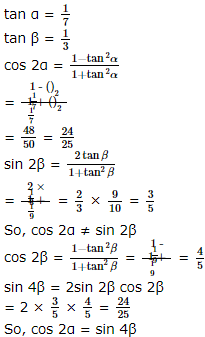SSC CPO Mock Test (Paper 1) - 5 - Question 137

A cone of height 9 cm with diameter of its base 18 cm is carved out from a wooden solid sphere of radius 9 cm. The percentage of the wood wasted is :

SSC CPO Mock Test (Paper 1) - 5 - Question 138

The volumes of two spheres are in the ratio of 64:27. The ratio of their surface areas is :

SSC CPO Mock Test (Paper 1) - 5 - Question 139

A can do a certain work in the same time in which B and C together can do it. If A and B together could do it in 10 days and C alone in 50 days, then B alone could do it in :

Detailed Solution for SSC CPO Mock Test (Paper 1) - 5 - Question 139

A = B + C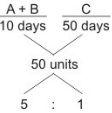⇒ A + B + C = 6 units
But A = B + C
⇒ 2(B + C) = 6 units
⇒ B + C = 3 units
⇒ B + C - C = 3 - 1 = 2 units
⇒ B = 2 units
Hence, B alone did it in:
50 2 = 25 days

SSC CPO Mock Test (Paper 1) - 5 - Question 140

The value of cot A + tan (180º+A)+tan(90º+A)+tan (360º-A) is

SSC CPO Mock Test (Paper 1) - 5 - Question 141

A cylindrical tank of diameter 35 cm is full of water. If 11 litres of water is drawn off, the water level in the tank will drop by :

SSC CPO Mock Test (Paper 1) - 5 - Question 142

Production of two Companies A and B over the years (Production in Lakh units)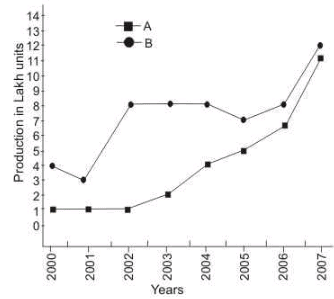For Company A, what is the percent decrease in production from 2000 to 2001 ?

SSC CPO Mock Test (Paper 1) - 5 - Question 143

Production of two Companies A and B over the years (Production in Lakh units)In 2007, the production of Company B is approximately what percent of that of in 2006 ?

SSC CPO Mock Test (Paper 1) - 5 - Question 144

Production of two Companies A and B over the years (Production in Lakh units)For Company A, in which year is the percentage increase/decrease in the production from the previous year the highest ?

SSC CPO Mock Test (Paper 1) - 5 - Question 145

Production of two Companies A and B over the years (Production in Lakh units)What is the difference in the total production of the Companies for the given years ?

SSC CPO Mock Test (Paper 1) - 5 - Question 146

These questions are to be answered on the bases of the following pie chart which gives the marks scored by a student in an examination in five subjects- English,Hindi,Mathematics,Science and Social Science. Assuming that the total marks obtained for the examination are 540 answer these questions.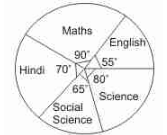The marks scored by the student in Hindi and Mathematics exceed the marks scored in English and Social Science by :

SSC CPO Mock Test (Paper 1) - 5 - Question 147

These questions are to be answered on the bases of the following pie chart which gives the marks scored by a student in an examination in five subjects- English,Hindi,Mathematics,Science and Social Science. Assuming that the total marks obtained for the examination are 540 answer these questions.he subject in which the student scored 22.2% marks is :

SSC CPO Mock Test (Paper 1) - 5 - Question 148

These questions are to be answered on the bases of the following pie chart which gives the marks scored by a student in an examination in five subjects- English,Hindi,Mathematics,Science and Social Science. Assuming that the total marks obtained for the examination are 540 answer these questions.The marks obtained in three subjects , English , Science and Social Science , are what percent of the total marks ?

SSC CPO Mock Test (Paper 1) - 5 - Question 149

These questions are to be answered on the bases of the following pie chart which gives the marks scored by a student in an examination in five subjects- English,Hindi,Mathematics,Science and Social Science. Assuming that the total marks obtained for the examination are 540 answer these questions.The marks obtained in Mathematics are what percent of the total marks ?

SSC CPO Mock Test (Paper 1) - 5 - Question 150

These questions are to be answered on the bases of the following pie chart which gives the marks scored by a student in an examination in five subjects- English,Hindi,Mathematics,Science and Social Science. Assuming that the total marks obtained for the examination are 540 answer these questions.The aggregate marks obtained by the students averaged over the 5 subject is :

SSC CPO Mock Test (Paper 1) - 5 - Question 151

Find the antonym of DESTINY

SSC CPO Mock Test (Paper 1) - 5 - Question 152

Find the antonym of ARTIFICIAL

SSC CPO Mock Test (Paper 1) - 5 - Question 153

Find the antonym of DETESTS

SSC CPO Mock Test (Paper 1) - 5 - Question 154

Find the antonym of BASE

SSC CPO Mock Test (Paper 1) - 5 - Question 155

Find the Antonym of HESITATE

SSC CPO Mock Test (Paper 1) - 5 - Question 156

Choose the correctly spelt word.

SSC CPO Mock Test (Paper 1) - 5 - Question 157

Choose the correctly spelt word.

SSC CPO Mock Test (Paper 1) - 5 - Question 158

Choose the correctly spelt word.

SSC CPO Mock Test (Paper 1) - 5 - Question 159

Choose the correctly spelt word.

SSC CPO Mock Test (Paper 1) - 5 - Question 160

Choose the correctly spelt word.

SSC CPO Mock Test (Paper 1) - 5 - Question 161

Fill in the blank with appropriate word.
He is in the habit of.........his head whenever anything goes wrong.

SSC CPO Mock Test (Paper 1) - 5 - Question 162

Fill in the blank with appropriate word.
There was no.........in the railway compartment for additional passengers.

SSC CPO Mock Test (Paper 1) - 5 - Question 163

Fill in the blanks with appropriate word .

Mass migration has produced a huge world wide economy of its own which has ___B1___ so fast during the past few years that the figures have ___B2___ experts. Last year remittances sent home by migrants were expected to ___B3___ \$232 billion according to the World Bank which ___B4___ these figures. ___B5___ though the flow of remittances is to alleviate the plight of the migrant’s family it cannot on its own lift entire nations out of poverty. Those who study the ___B6___ of remittances argue that the money allows poor countries to put off basic decisions of economic management like ___B7___ their tax collection systems and building schools. Remittances to poor countries can also ___B8___ fact that they do not produce much at home. The challenge is how to find programmes that ___B9___ the benefits of remitted cash while ___B10___ some of its downside.

Appropriate word at B1 is

SSC CPO Mock Test (Paper 1) - 5 - Question 164

Fill in the blanks with appropriate word .

Mass migration has produced a huge world wide economy of its own which has ___B1___ so fast during the past few years that the figures have ___B2___ experts. Last year remittances sent home by migrants were expected to ___B3___ \$232 billion according to the World Bank which ___B4___ these figures. ___B5___ though the flow of remittances is to alleviate the plight of the migrant’s family it cannot on its own lift entire nations out of poverty. Those who study the ___B6___ of remittances argue that the money allows poor countries to put off basic decisions of economic management like ___B7___ their tax collection systems and building schools. Remittances to poor countries can also ___B8___ fact that they do not produce much at home. The challenge is how to find programmes that ___B9___ the benefits of remitted cash while ___B10___ some of its downside.

Appropriate word at B2 is

SSC CPO Mock Test (Paper 1) - 5 - Question 165

Fill in the blanks with appropriate word .

Mass migration has produced a huge world wide economy of its own which has ___B1___ so fast during the past few years that the figures have ___B2___ experts. Last year remittances sent home by migrants were expected to ___B3___ \$232 billion according to the World Bank which ___B4___ these figures. ___B5___ though the flow of remittances is to alleviate the plight of the migrant’s family it cannot on its own lift entire nations out of poverty. Those who study the ___B6___ of remittances argue that the money allows poor countries to put off basic decisions of economic management like ___B7___ their tax collection systems and building schools. Remittances to poor countries can also ___B8___ fact that they do not produce much at home. The challenge is how to find programmes that ___B9___ the benefits of remitted cash while ___B10___ some of its downside.

Appropriate word at B3 is